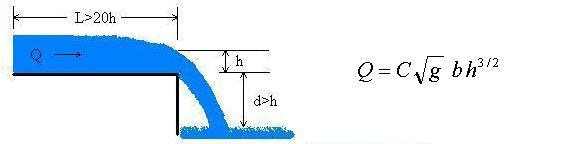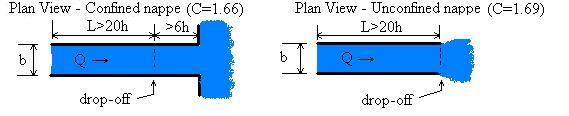Open Channel Flow Measurement in Rectangular Channel Waterfall using End Depth Method

Compute discharge, depth, or width for a horizontal rectangular channel with a sudden drop-off (free overflow). Waterfall.

 Select channel: Confined nappe Unconfined nappe ©2014 LMNO Engineering, Solve for: Discharge Depth Width Research, and Software, Ltd. www.LMNOeng.com Discharge, Q: m3/s (cms) ft3/s (cfs) U.S. gal/min (gpm) U.S. gal/day (gpd) Acre-ft/yr Water Depth, h: cm m inch ft Channel Width, b: cm m inch ft

Units in waterfall calculator: cm=centimeter, cfs=cubic foot per second, cms=cubic meter per second, ft=foot, gal=gallon (U.S.), m=meter, min=minute, s=second, yr=year

Rectangular Open Channel Waterfall End Depth Method Drawings and EquationIntroduction to Rectangular End Depth Method for Waterfalls
The end-depth method for determining discharge is very useful since channels are easily constructed to have a free overfall, and natural channels (waterfalls in rivers and streams) often have this configuration. Due to the minimal installation costs (if any), flow rate measurement using the end depth method is much less expensive than installing a weir or flume. The equation shown above for determining discharge in a waterfall in a rectangular open channel is from the International Organization of Standards publication 3847 dated 1977 (ISO, 1977). For the equation to give an accurate discharge measurement, there are several criteria that should be met:
• Clear water.
• Rectangular channel for a distance at least 20h upstream of the drop-off (aka. overflow or overfall).
• Channel should be horizontal although a slight slope is okay.
• Channel should be smooth such as would be provided by a neat cement finish.
• Flow should be sub-critical upstream of the drop-off.
• The drop, d, should be greater than h.
• The channel width, b, should be greater than 0.3 m (11.8 inch).
• The end depth, h, should be greater than 0.04 m (1.6 inch).
• For a confined nappe, the channel should be rectangular for a distance of greater than 6h downstream of the drop-off, and the nappe should be ventilated so that the water does not cling to the drop off.

If the criteria are met and h is measured carefully, the accuracy of the discharge measurement is 2% for a horizontal channel and up to 7% for a slightly sloping channel (ISO, 1977).

Variables for Rectangular End Depth Waterfall Equation [L] indicates length units. [T] indicates time units.
b = Channel width [L]. Must be greater than 0.3 m (11.8 inch) for the equation to be reliable.
C = Coefficient of discharge. C=1.66 for confined nappe. C=1.69 for unconfined nappe.
d = Drop elevation [L]. Elevation difference between bottom of channel and tail water. Must be at least h for the equation to be reliable.
g = Acceleration due to gravity=9.8066 m/s2.
h = Measured water depth at end of channel (i.e. at the drop-off) [L]. Must be greater than 0.04 m (1.6 inch) for the equation to be reliable.
L = Straight length upstream of drop-off [L]. Must be at least 20h for the flow to be well-established.
Q = Discharge [L3/T]. Also known as flowrate.

Error Messages for Waterfall Discharge Calculation
"h and b must be >0." Check your input. h or b was entered as 0.0 or negative.
"Q and b must be >0." Check your input. Q or b was entered as 0.0 or negative.
"Q and h must be >0." Check your input. Q or h was entered as 0.0 or negative
"Need b>0.3 m for accuracy." b (channel width) was entered or computed as ≤ 0.3 m. The calculation finished, but the equation is not accurate for such a narrow channel.
"Need h>0.04 m for accuracy." h was entered or computed as ≤ 0.04 m. The calculation finished, but the equation is not accurate for such a small depth.

References for Waterfall End Depth Flow Calculation
International Organization of Standards (ISO 3847). 1977. Liquid flow measurement in open channels by weirs and flumes - End depth method for estimation of flow in rectangular channels with a free overfall. Reference number: ISO 3847-1977(E).

LMNO Engineering, Research, and Software, Ltd.
7860 Angel Ridge Rd.   Athens, Ohio 45701  USA   Phone: (740) 707-2614
LMNO@LMNOeng.com    http://www.LMNOeng.com

To: